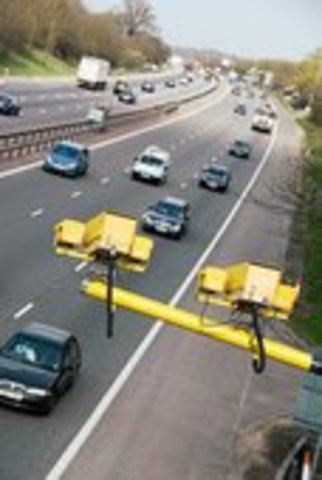• # speed isspeed is how fast an object is moving
formula speed = distance / time
m m | 1 m
__ = _______ = ___

s 1 | s s
• # disatance• # background time• # formula

speed = distance / time
• # units for speed

speed = distance / seconds
distance = meters
time = seconds
• # practice

distance = 5 meters
time = 2 seconds
speed = find speed in mters/ seconds